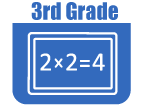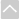Review Activities and Games

These resources are intended for review and practice after initial instruction of the standards.

Strand: MATHEMATICAL PRACTICES (3.MP)Strand: OPERATIONS AND ALGEBRAIC THINKING (3.OA)
Represent and solve problems involving multiplication and division within 100 (Standards 3.OA.1–4 and Standard 3.OA.7)
• Arithmetic Four
In this game for two players, students solve problems involving whole number and integer addition, subtraction, multiplication, and division.
• Factor Trail Game
In this lesson students will practice identifying the integer factors of numbers up to 100.
They demonstrate understanding of the properties of multiplication and the relationship between multiplication and division (Standards 3.OA.5–6)
• Arithmetic Four
In this game for two players, students solve problems involving whole number and integer addition, subtraction, multiplication, and division.
Represent and solve problems involving multiplication and division within 100 (Standards 3.OA.1–4 and Standard 3.OA.7)
• Arithmetic Four
In this game for two players, students solve problems involving whole number and integer addition, subtraction, multiplication, and division.
• Factor Trail Game
In this lesson students will practice identifying the integer factors of numbers up to 100.
Students use the four operations to identify and explain patterns in arithmetic (Standards 3.OA.8–9)Strand: NUMBER AND OPERATIONS IN BASE TEN (3.NBT)
Use place value understanding and properties of operations to perform multi-digit arithmetic. A range of algorithms may be used (Standards 3.NBT.1–3).Strand: NUMBER AND OPERATIONS - FRACTIONS (3.NF)
Develop understanding of fractions as numbers. Denominators are limited to 2, 3, 4, 6, and 8 in third grade. (3.NF.1-3)
• Comparing Fractions with Lines
This lesson will help students simplify fractions, compare and order them on a number line, and estimate their value.
• Equivalent Fractions Finder
Students doing this lesson will visually experiment with the relationship between the value of fractions and areas within a square or a circle. In doing so they will practice simplifying fractions and compare fractions.
• Equivalent Fractions Pointer
This lesson will help students understand how to simplify and compare fractions as well as place them in order on a number line.
• Single Fraction Finder
In this lesson students will practice dividing a whole into a fraction and visualize fractions on a number line.
• Single Fraction Pointer
This activity was designed to help students better understand fractions by practicing dividing a whole into a fraction and visualizing fractions on a number line.
• What Fraction of this Shape is Red?
This Teaching Channel video shows how students can explore part and whole by creating pattern block designs. (4 minutes)Strand: MEASUREMENT AND DATA (3.MD)
Solve problems involving measurement and estimation of intervals of time, liquid volumes, and masses of objects. (3.MD.1–2)
• Elapsed Time Two
This lesson can be used to help students understand how to read a clock, as well as understand addition and subtraction with time.
Represent and interpret data (Standards 3.MD.3– 4)
• Histogram
This activity will help students understand histograms by allowing them to make their own.
• IXL Game: Interpret Pictographs
This game helps the third grader draw a scaled picture graph and a scaled bar graph to represent a data set with several categories. This is just one of many online games that supports the Utah Math core. Note: The IXL site requires subscription for unlimited use.
Understand concepts of area and relate area to multiplication and addition (Standards 3.MD.5–7)
• Area (elementary)
In this lesson students will find the area of rectangular shapes and irregular shapes with right angles.
• Area Explorer
The applet in this activity generates a random geometric figure and allows the student to test their understanding of how to find the volume of an area.
• India's Bathroom Tiles
Students are given a word problem about tiling a bathroom. This task allows students to use the concept of "tiling" as an entry point to linking area with multiplication. Ideally, they would be given foam or plastic tiles to actually practice putting the tiles on the space without gaps or overlaps.
• Shape Builder
This activity allows the student to explore area and perimeter by either allowing the computer to randomly draw a shape on a grid or build the shape on the grid.
• Shape Explorer
The applet contained in this lesson allows the student to test his or her skill at calculating the area and perimeter of a various figures.
Recognize perimeter as an attribute of plane figures and distinguish between linear and area measures (Standard 3.MD.8)
• Area Explorer
The applet in this activity generates a random geometric figure and allows the student to test their understanding of how to find the volume of an area.
• Perimeter
This lesson plan and activities will help students understand the concept of perimeter.
• Perimeter (elementary)
This lesson will help students find the perimeter of rectangular shapes and irregular shapes with right angles.
• Shape Builder
This activity allows the student to explore area and perimeter by either allowing the computer to randomly draw a shape on a grid or build the shape on the grid.
• Shape Explorer
The applet contained in this lesson allows the student to test his or her skill at calculating the area and perimeter of a various figures.Strand: GEOMETRY (3.G)
Reason with shapes and their attributes. (Standards 3.G.1–2)
In this lesson the student is introduced to parallelograms, rectangles, and trapezoids and practices creating various types of quadrilaterals.
• Floor Tiles
This lesson will help students identify and examine symmetry in geometric figures as well as describe and classify polygons.
• Perimeter
This lesson plan and activities will help students understand the concept of perimeter.
• Sets and The Venn Diagram (Beginner)
By introducing students to the idea of a set, this lesson will help students learn about the vocabulary of sets and Venn diagrams, as well as how to arrange things in a Venn diagram.
• Sets and the Venn Diagram
This lesson is designed to help students understand the ideas surrounding sets and Venn diagrams.
• Single Fraction Pointer
This activity was designed to help students better understand fractions by practicing dividing a whole into a fraction and visualizing fractions on a number line.
• Venn Diagram Shape Sorter
This lesson is designed to introduce young students to the concept Venn diagrams and explore different kinds.
• What Fraction of this Shape is Red?
This Teaching Channel video shows how students can explore part and whole by creating pattern block designs. (4 minutes)The Online Core Resource pages are a collaborative project between the Utah State Board of Education and the Utah Education Network. If you would like to recommend a high quality resource, contact Trish French (Elementary) or Lindsey Henderson (Secondary). If you find inaccuracies or broken links contact resources@uen.org.